# Guide to Autoregressive ModelsSome stock prices follow a consistent trend while others don't. Again, some of the company's stock price recover after a decline. Even though these questions doesn’t seem connected, they do have a common solution - time series models.

The values of past and present time series often resemble one another. As a result, such data are subject to autocorrelation. Therefore, we can often roughly predict a product's valuation tomorrow by knowing its current price. Hence, we will discuss the autoregressive model in this tutorial that reflects this correlation.

## What is an autoregressive model?

An autoregressive (AR) model forecasts future behavior based on past behavior data. This type of analysis is used when there is a correlation between the time series values and their preceding and succeeding values.

Autoregressive modeling uses only past data to predict future behavior. Linear regression is carried out on the data from the current series based on one or more past values of the same series.

AR models are linear regression models where the outcome variable (Y) at some point of time is directly related to the predictor variable (X). In AR models, Y depends on X and previous values for Y, which is different from simple linear regression.

### Interpreting the autoregressive model

We know that in the autoregressive model, or AR for short, current values is predicted based solely on previous values. Basically, this is a linear model in which current period values is derived from past outcome sums multiplied by a numeric factor.

Using AR(p), we can consider the number of lagged values we want to include in the model, where p represents the model's order. A simple autoregressive model, also known as an AR(1), would look like this, for example, if X is a time-series variable:

Xt = C + ϕ1Xt-1 + ϵt

Let's break down each part of this equation to understand the concept.

What is Xt-1?

In the first place, Xt-1 represents the previous period's value of X.

Let’s elaborate.

“t” represents today, while “t-1” represents last week. As a result, Xt-1 reflects last week's value.

What is ϕ1?

The coefficient ϕ1 represents the numeric constant multiplied by the lagged variable (Xt-1). In other words, it represents the future portion of the previous value.

Try to maintain these coefficients between -1 and 1. The reason for this is as follows.

When the absolute value of the coefficient exceeds 1, it will explode exponentially over time. Initially, this concept may seem confusing. Here is an example from math.

Consider that we have a time series with 1000 observations, and ϕ1 = 1.3 and C=0.

Then, X2 = 0 + 1.3 x 1

Since X3 = 1.3 x 2, by substituting (1.3 x 1) for X2 and obtain X3 = 1.3(1.3 x 1) = 1.32 X1. In addition, the longer the period (e.g. X50), the coefficient significantly increases (1.349 x 1).

When we reach the 1000th period, we can get X1000 = 1.3999 x 1. As a result, the values increase continuously and end up much higher than what they began with. It appears that this is not a reliable method of predicting the future.

What is ϵt?

It's time to take a closer look at the other part of the equation, which is ϵt.

This value is known as residual, representing the difference between our period t prediction and the correct value (ϵt = yt - ŷt).

As a result of this pattern getting captured by the other mandatories in the model, the residuals are usually unpredictable.

## Understanding autoregressive models

It is a common belief that past values influence current values in autoregressive models. Hence, this is why the statistical technique is widely getting used to analyze natural phenomena, economic processes, and other processes that change over time.

Many regression models use linear combinations of predictors to forecast a variable. In contrast, autoregressive models use the variable's past values to determine the future value.

AR(1) autoregressive processes depend on the value immediately preceding the current value. Alternatively, AR(2) uses the previous two values to calculate the current value. While AR(0) processes white noise, which does not depend on terms.

The least squares method gets used to calculate coefficients with these variations. Technical analysts make predictions about security prices using these forecasting concepts and techniques.

Since autoregressive models predict prices only based on past information, it assumes that the fundamentals will remain the same. As a result, predictions may be inaccurate or surprising if the underlying forces change, particularly when an industry is rapidly transforming in technological ways.

## Example of an autoregressive model

The autoregressive model assumes that the past value affects the current values. For instance, investors using autoregressive models for stock price forecasts should assume that recent market transactions will influence new purchasers' and sellers' decisions when making and accepting offers for security.

Generally, this assumption holds, but it is not always the case. Many financial firms held large portfolios of mortgage-backed securities before the 2008 financial crisis, which posed many risks to investors.

A financial stock investor using an autoregressive model at that time would have had good reason to believe that prices in that sector would stay stable or rise for the predictable future.

As soon as the investors realized that many financial institutions were in danger of collapsing, they became less concerned with recent stock prices and became more concerned with their underlying risks.

Thus, financial stocks were quickly revalued to a much lower level, making autoregressive models useless. In an autoregressive model, it is crucial to note that a one-time shock can permanently affect the variables. Thus, today's autoregressive models bear the scars of the financial crisis.

## Autoregressive model benefits

• Advantage of this model is that you can tell if there is a lack of randomness by using the autocorrelation function.

• Additionally, it is capable of forecasting recurring patterns in data.

• It is also possible to predict outcomes with less information using self-variable series.

## Autoregressive model limitations

There are several limitations associated with this method:

• The autocorrelation coefficient must be at least 0.5 in this case for it to be appropriate. This means that if it's less than 0.5, the prediction result will be inaccurate.

• It is usually used while predicting things associated with economics based on historical data. Something that is significantly affected by social factors. It is highly recommended to use the vector autoregressive model instead. The reason being a single model can be used to predict multiple time series variables at the same time.

## What is time series forecasting?

In time series forecasting, time series data is analyzed through statistics and mathematical modeling to predict and inform strategic decisions.

It's neither an exact prediction nor is it possible to predict with 100% accuracy, particularly while dealing with frequently changing variables and some beyond our control variables.

However, forecasting can provide insight into the likelihood of certain outcomes. Generally, a more extensive dataset leads to more accurate forecasting.

Predictions and forecasts are generally synonymous, but there is a notable difference between them. Prediction refers to data at a general future point in time, whereas forecasting focuses on data at a specific future point when it occurs.

The analysis of time series is often combined with series forecasting. In time series analysis, models are developed to understand the underlying causes of the data. By analyzing outcomes, you can understand "why" they occur. As a result, forecasting takes the next step of extrapolating the future from the knowledge derived from the past.

### Applications of time series forecasting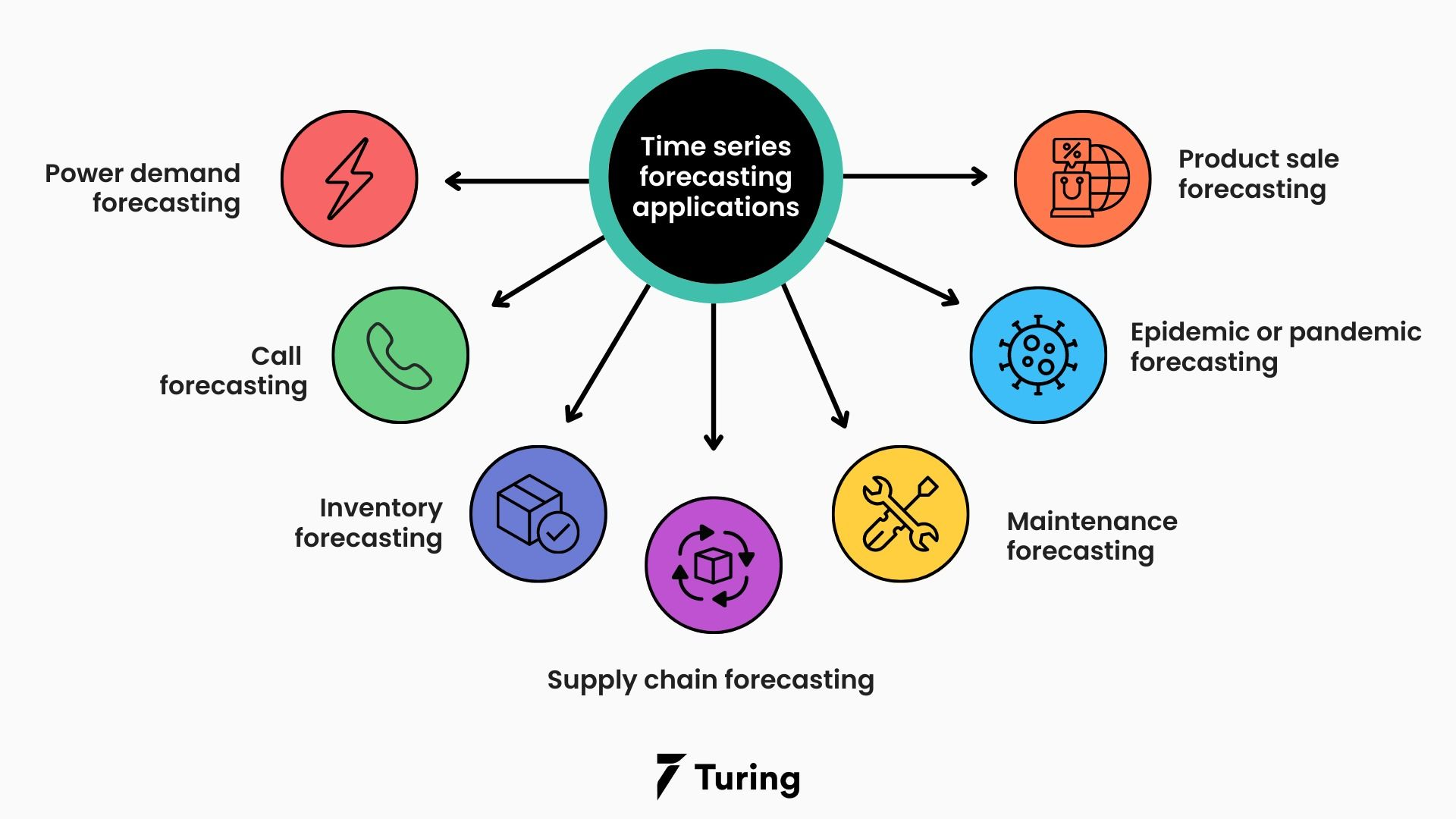From sales forecasting to weather forecasting, time series models have a wide range of applications.

A time series model is one of the most effective methods of forecasting when there is uncertainty about the future.

A time series forecast plays a crucial role in every category of the business decision. Here are some examples:

• Power demand forecasts help determine whether another power plant should get built in the next five years.
• Scheduling of calls for the next week is done based on the call volume forecast.
• To forecast inventory requirements so as to stock items to meet demand.
• A supply chain management forecast is used to optimize fleet management and other aspects of the supply chain.
• To minimize downtime and maintain safety standards by predicting equipment failures and maintenance requirements.
• The outbreak of epidemic or pandemic is controlled by forecasting infection rates.
• Analyzing customer ratings and forecasting product sales.

Different forecasts involve different time horizons and can depend on the circumstances.

## What is time series data?

Time series data is gathered by observations made over a long period of time. You would always plot the points on a graph with time as one of the axes. A time series metric refers to a metric that tracks data over time.

For example, in a store inventory, a metric could be the amount of inventory sold every day. Because time is a constituent of everything observable, time series data is everywhere. Time series data is constantly streaming out of sensors and systems throughout our increasingly instrumented world.

There are many applications for such data across many industries. To understand this, let's look at some examples. An example of a time series analysis would be as follows:

• Electrical activity in the brain
• Rainfall measurements
• Stock prices
• Number of sunspots
• Annual retail sales
• Monthly subscribers
• Heartbeats per minute

### What is an example of time series data?

The goal of time series analysis is to understand how a variable's value changes over time. Here are examples of the common use of time series analysis in real-life situations.

Example 1: Heart rate

A medical application of time series analysis is monitoring the heart rate of patients taking certain medications to ensure that it does not fluctuate too wildly throughout the day.

Example 2: Retail sales

To analyze trends in total sales over time, many retail stores use time series analysis. The time series analysis is particularly useful for analyzing sales trends over a month, a season, or a year.

Retail stores can accurately predict the amount of inventory and staff they will need throughout a particular period by using this method.

Example 3: Stock prices

Stock traders use time series analysis to understand patterns in various stock prices so that they can gain a better understanding of them.

The plot of time series is particularly useful for stock analysts and traders because it allows them to determine the trend and direction of a stock price.

## What is vector autoregression(VAR)?

Vector autoregressive (VAR) models are multivariate time series models that relate current observations of one variable to past observations of that variable and other variables.

Variable feedback is a characteristic of VAR models, unlike univariate autoregressive models.

An example of this is to show how real GDP affects policy rate and how policy rate affects real GDP. To perform a complete VAR analysis, a multi-step process is required, including:

• Specifying and estimating a VAR model.
• Checking and revising the model (as needed).
• Forecasting.
• Structural analysis.

The VAR model belongs to a class of multivariate linear time series models known as vector autoregression moving average (VARMA) models.

The multivariate linear time series model is generally useful for the following purposes:

• Simulating the movements of many stationary time series at the same time.
• A system-based method to measure delayed effects of response variables.
• The use of exogenous series to analyze the effects on a system's variables. For example, examine whether a recent tariff has affected several econometric series.
• Forecasting the response variables simultaneously.

## Vector autoregression model in Python

``````import numpy as np
import pandas as pd
import statsmodels.api as sm
from statsmodels.tsa.api import VAR
from statsmodels.tsa.base import  datetools

``````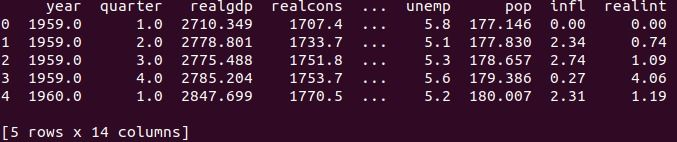``````mdata = mdata[['year','quarter']].astype(int)
mdata = mdata[['year','quarter']].astype(str)
quarterly = mdata["year"] + "Q" + mdata["quarter"]
quarterly = datetools.dates_from_str(quarterly)
quarterly
``````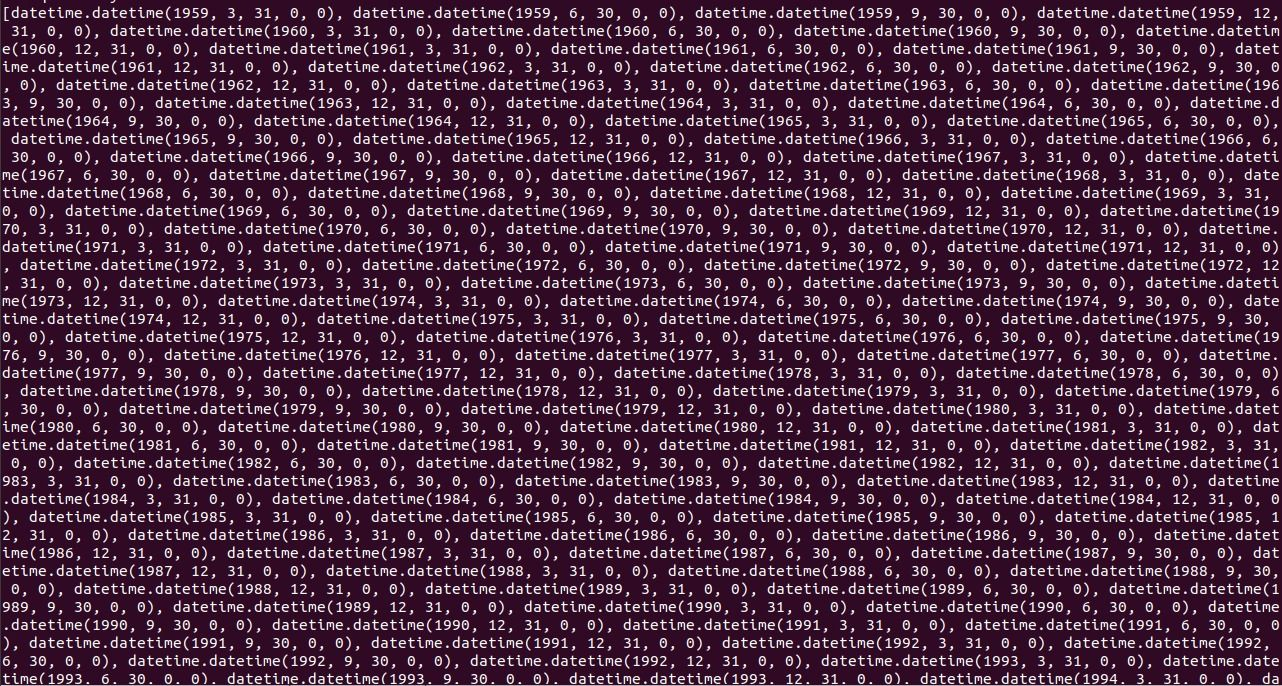``````mata = mdata[['realgdp','realcons','realinv']]
mdata.index = pd.DatetimeIndex(quarterly)
``````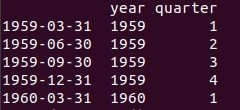``````mdata.plot()
``````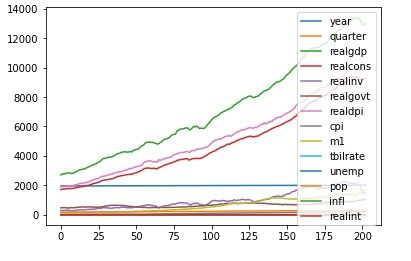``````data = np.log(mdata).diff().dropna()
data.plot()
``````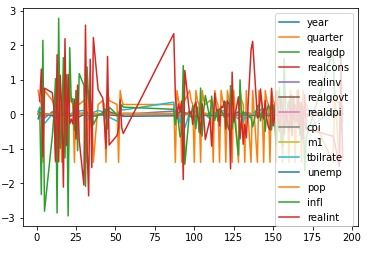## Conclusion

The autoregressive model predicts the future based on the previous data. A lot of technical analysts use them to predict future security prices. In this type of analysis, time series values are correlated with their predecessors and successors.

The central idea behind autoregressive models is to predict the next value in a vector time series by using the squared coefficient of previous values.

The autoregressive models are the statistical models that have worked well for us in various applications, such as time series forecasting and financial forecasting. They are also used to create models based on time series data.

## Author

•### Turing

Author is a seasoned writer with a reputation for crafting highly engaging, well-researched, and useful content that is widely read by many of today's skilled programmers and developers.

AR(1) is stationary only if |φ| < 1 or −1 <φ< 1. This is a non-stationary explosive process.

As a result of combining all the inequalities, we can find a region bounded by the lines φ2 =1+ φ1; φ2 = 1 − φ1; φ2 = −1. This is the region where the AR(2) process is stationary.

Therefore, AR, MA, and ARMA are either stationary or non-stationary depending on the parameters

AR and MA models differ primarily in how they correlate time series objects at different points in time. MA models have zero covariance between x(t) and x(t-n).

In the AR model, however, the correlation between x(t) and x(t-n) gradually declines as n increases. It means that the moving average(MA) model uses the errors from past forecasts rather than past forecasts to predict future values.

On the other hand, an autoregressive model(AR) uses past forecasts for future predictions.

The autoregressive model predicts the future based on the past data. A lot of technical analysts use them to predict future security prices. In autoregressive models, the future gets implicitly considered to be similar to the past.### Press

What's up with Turing? Get the latest news about us here.### Blog